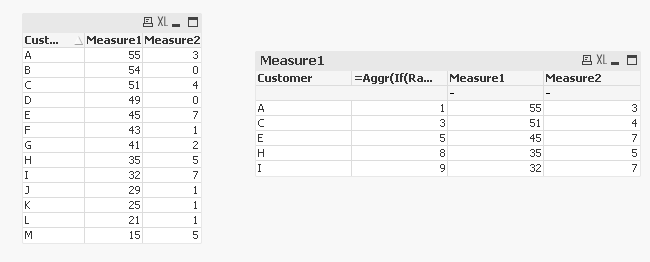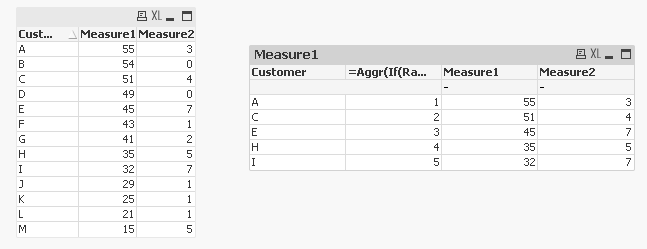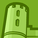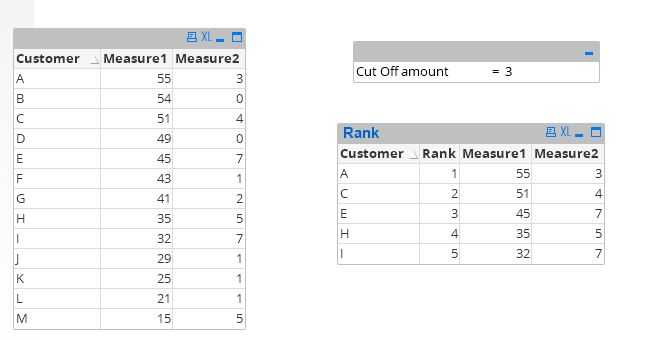# QlikView App Dev

Discussion Board for collaboration related to QlikView App Development.

Announcements
Our next Qlik Insider session will cover new key capabilities. Join us August 11th REGISTER TODAY
cancel
Showing results for
Did you mean:Creator

## Rank in Qlikview based on Cut off Value

Hi,

I have a report to show show the Top N customers.

The logic is to Show Top N by "Measure1" where "Measure2" is greater than a Cut off Amount.

Users can key in this cutoff amount in an Input Box.

I use the formula below :

=if(aggr((\$(vMeasure2)),customer) >= \$(vCutoff),IF((Aggr(Rank((\$(vMeasure1)),1,1),customer)) <= 20,(Aggr(Rank((\$(vMeasure1)),1,1),customer))))

The issue is the formula gets me the Top 20 customer by Measure1 and then filters the Measure2 to be greater  than or equal to cutoff value.

So if out of the list of 20 if only 3 customers have Measure2 greater than or equal to cutoff ,it shows only those 3 in the report.

But the requirement is to filter all customers with Measure2 greater then or equal cutoff and then Rank and show all 20 customers.

I tried to use a Set analysis for this,but gives error.

E.g. : Suppose if I want to see Top 5 and cutoff vale = 2

Attached is the example for the Expected and Actual results.

Thanks

9 RepliesMVP

May be this:

=Aggr(If(Rank(If(Measure2 > vCutOff, Measure1), 1, 1) <= 5, Rank(Measure1, 1, 1)), Customer)MVP

Or better yet, try this:

=Aggr(If(Rank(If(Measure2 > vCutOff, Measure1), 1, 1) <= 5, Rank(If(Measure2 > vCutOff, Measure1), 1, 1)), Customer)Master III

Check this also with initial filtering using set expression-MVP

Hey Digvijyay -

I am on Apple Mac computer and cannot open your qvw file. Would you be able to share a image and dimension and expression details to show what have you done?

Thanks,

SunnyCreator
Author

Hi Sunny,

Thanks for the suggestion,but it does not serve the purpose the Tank is still no in order of 1,2,3..,there is a break in between.

Please suggest if any other solution.

Thanks !Master III

Hi Sunny,

PFB the details -Rank exp - Rank(Measure1,1,1)

Measure1 - if(rank(Aggr(only({<Measure2={">=\$(vTop)"}>}Measure1),Customer),1,1)<=5, Measure1)

Measure2 - if(rank(Aggr(only({<Measure2={">=\$(vTop)"}>}Measure1),Customer),1,1)<=5, Measure2)

Regards,

DJMVP

Thanks Digvijay for sharing this. I think these expressions can be further simplified

2) Only({<Customer = {"=Rank(Only({<Measure2={'>=\$(vTop)'}>}Measure1),1,1) <= 5"}>}Measure1)

3) Only({<Customer = {"=Rank(Only({<Measure2={'>=\$(vTop)'}>}Measure1),1,1) <= 5"}>}Measure2)Master III

Perfect! Thanks.MVP

Prachi -

Where do you see a break in Rank? Are you seeing my updated sampleCommunity Browser